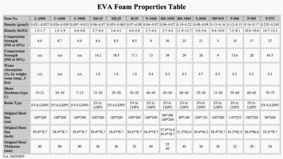### COMPRESSIVE STRENGTH

Compressive Strength, is the force necessary to produce a 25% (or any given percentage) compression over the entire top area of the foam specimen.
Calculation:

Calculate the 25% compression deflection force, per unit area of specimen, expresssed as kilopascals (or pound-force per square inch), as follows:

CD = F / A

where:
CD = compression deflection force per unit of specimen area, kPa (psi).
F = force required to compress the specimen 25% of the thickness as measured. N (lbf).
A = specimen compression contast surface area, m^2 (in^2).More technical data of EVA foam material